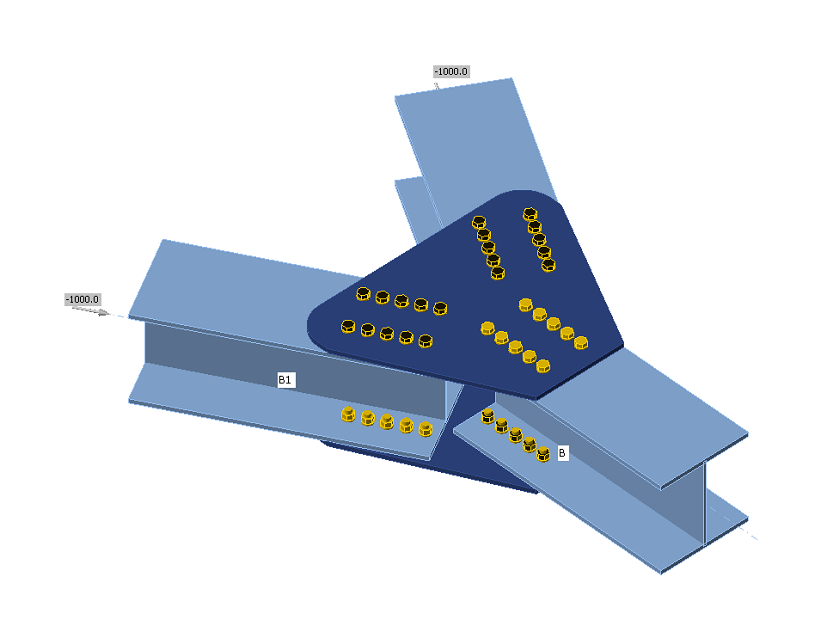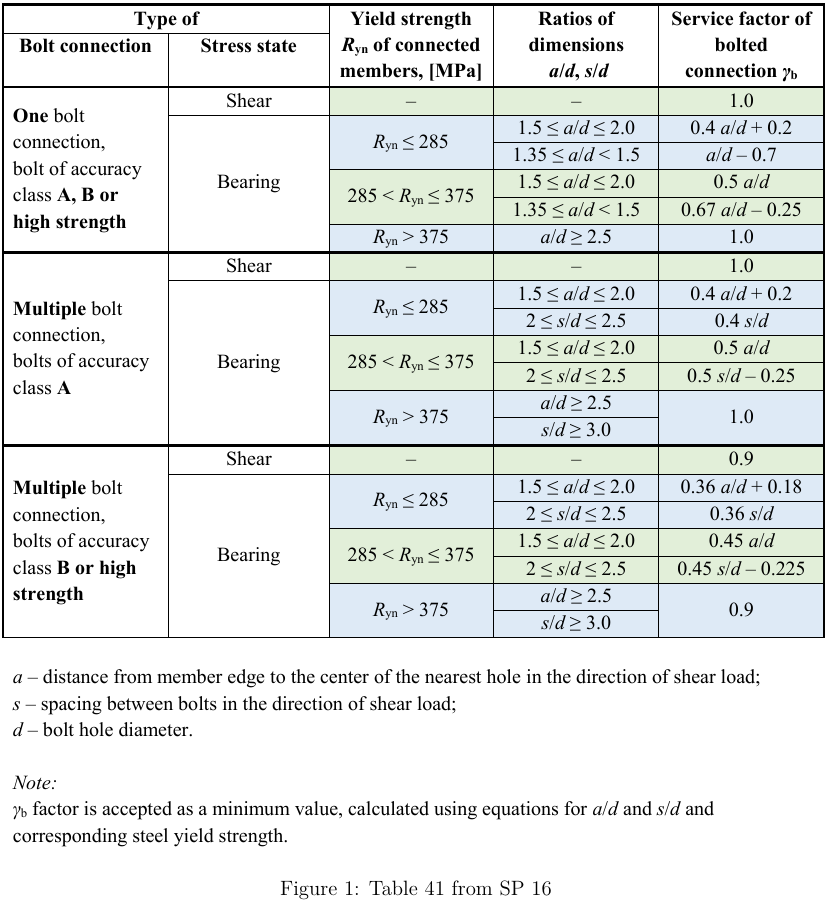### Choose language# Code-check of bolts and preloaded bolts according to Russian standards

$$BoltsBolts are checked according to SP 16, Cl. 14.2. The tensile and shear force in each bolt is determined by finite element analysis. Prying forces are determined by finite element analysis and taken into account. Each shear plane is checked individually. Ply in bearing is checked against the sum of shear forces at nearby planes.Bolt in shearA bolt subject to a design shear force is designed according to Cl. 14.2.9 and shall satisfy:$N_s \le N_{bs} = R_{bs} A_b \gamma_b \gamma_c$where: Ns – shear force in one plane of a bolt Nbs – bolt shear resistance Rbs – design shear strength of a bolt – SP 16, Table 5 Ab – bolt gross section area γb – service factor of bolt joint – SP 16, Table 41 – γb = 1.0 for single bolting and multibolting with accuracy class A, γb = 0.9 for multibolting and accuracy class B and high strength bolts (Rbun ≥ 800 MPa) γc – service factor – SP 16, Table 1, editable in Code setup Rbyn [MPa]Rbs [MPa] $$R_{byn} \le 300$$$$0.42 \cdot R_{bun}$$ $$300 < R_{byn} \le 400$$$$0.41 \cdot R_{bun}$$ $$400 < R_{byn} \le 936$$$$0.40 \cdot R_{bun}$$ $$936 > R_{byn}$$$$0.35 \cdot R_{bun}$$ Each shear plane is checked individually.Bolt in tensionA bolt subject to design tension force is designed according to SP 16, Cl. 14.2.9 and shall satisfy:$N_t ≤ N_{bt} = R_{bt} A_{bn} \gamma_c$where: Nt – tensile force in a bolt Nbt – bolt tensile resistance Rbt – design tensile strength – SP 16, Table 5 Abn – net cross-sectional area of a bolt γc – service factor – SP 16, Table 1, editable in Code setup Rbun [MPa]Rbt [MPa] $$R_{bun} < 830$$$$0.45 \cdot R_{bun}$$ $$830 \le R_{bun} < 1040$$$$0.54 \cdot R_{bun}$$ $$R_{bun} \ge 1040$$$$0.70 \cdot R_{bun}$$ Bolt subject to combined shear and tensionA bolt loaded in shear and tensile forces at the same time is designed according to SP 16, Cl. 14.2.13 and shall satisfy:$\sqrt{\left ( \frac{N_t}{N_{bt}} \right ) ^2 + \left ( \frac{N_s}{N_{bs}} \right ) ^2} \le 1.0$where: Nt – tensile force in a bolt Nbt – bolt tensile resistance Ns – shear force in one plane of a bolt Nbs – bolt shear resistance Bolts in bearingA plate subject to a bearing force due to a bolt in shear is designed according to SP 16, Cl. 14.2.9 and shall satisfy:$N_s ≤ N_{bp} = R_{bp} d_b t \gamma_b \gamma_c$where: Ns – shear force in a bolt acting on a ply Nbp – bearing resistance of a ply Rbp – bearing design strength; Rbp = 1.6 · Ru for accuracy class A and Rbp = 1.35 · Ru for accuracy class B – SP 16, Table 5 Run – ultimate strength of the connected element db – bolt diameter t – thickness of the plate γb – service factor of bolt joint – SP 16, Table 41 γc – service factor – SP 16, Table 1, editable in Code setup Each plate is checked individually and the worst case is shown. SP 16 does not show the service factor of bolted connection, γb for cases outside detailing limits. Therefore, the bearing check is not performed for such cases.Friction-type connectionsFor friction-type connections, the slip is required to be limited and checked according to SP 16, Cl. 14.3. These bolts should be also checked as bearing type for ultimate limit state after slip occurs. A bolt subjected to shear force shall satisfy:$N_s \le N_{bf} = Q_{bh} \gamma_b \gamma_c$where: Ns – shear force acting on one preloaded bolt and one friction plane Nbf – shear slip resistance of one preloaded bolt and one friction plane Qbh = Rbh Abn μ / γh – design slip resistance of one preloaded bolt and one friction plane Rbh = 0.7 · Rbun – design preload in the preloaded bolt – SP 16, Cl. 6.7 Rbun – ultimate tensile resistance of the bolt Abn – tensile stress area μ – frictional coefficient for preloaded bolts – SP 16, Table 42, editable in Code setup γh – coefficient in case of bolt tightening – SP 16, Table 42 Regular holes: static loading, Δ ≤ 4 mm; dynamic loading, Δ ≤ 1 mm: γh = 1.12 for μ ≥ 0.42 γh = 1.17 for 0.35 ≤ μ < 0.42 γh = 1.30 for μ < 0.35 Oversized holes: static loading, Δ > 4 mm; dynamic loading, Δ > 1 mm: γh = 1.70 for μ < 0.35 γh = 1.35 for μ ≥ 0.35 Δ – difference between diameters of bolt hole and of bolt γb – friction joint service factor – SP 16, Cl. 14.3.4 γc – service factor – SP 16, Table 1, editable in Code setup Static or dynamic loading can be set in Code setup. Number of bolts n$$\gamma_b$$ $$n < 5$$0.8 $$5 \le n < 10$$ 0.9 $$n \ge 10$$ 1.0 Number of effective interfaces, κ, is always equal to 1, because each interface is checked separately.According to SP 16, Cl. 14.3.6, for bolts in friction-type connections loaded by combined shear and tension, friction joint service factor, γb, is multiplied by:$\gamma_b = \gamma_b \cdot \left ( 1 - \frac{N_t}{P_b} \right )$where: Nt – tensile force in a bolt Pb = Rbh Abn – preload in a bolt Rbh = 0.7 · Rbun – design preload in the preloaded bolt – SP 16, Cl. 6.7 Abn – tensile stress area Friction-type connections should be also checked for ultimate limit state. The bolt type should be changed to bearing – tension/shear interaction, the loads increased appropriately and the joint should be checked again.$$